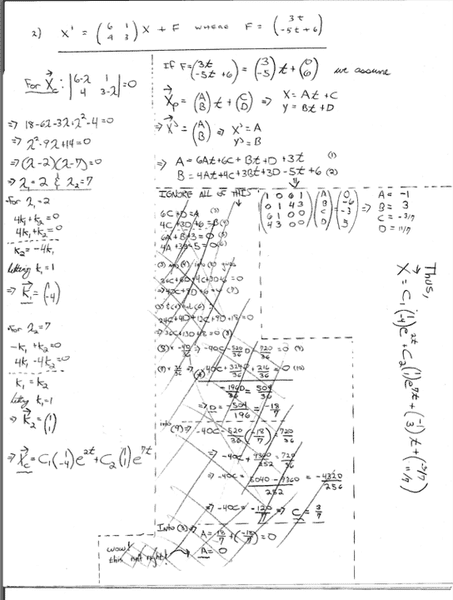# What the Hell. Differential Eq

[SOLVED] What the Hell. Differential Eq

Okay, so I used Method of undetermined coefficients for this one. I got a solution that when differentiated and plugged back in almost works, bit is off by a little.When I plug back into y'=4x+3y+(-5t+6)
I get y'=...+9 not 6. So I have a feeling it has to do with my D=11/7 term... but I have no idea what.

Anyone see what I am doing wrong? Is my matrix incorrect? Or my assumption of Xp? Or neither?

Thanks

Sorry, I forgot to change the matrix...this is what I used to get A,B,C,D

$$\left[\begin{array}{cccc}6 & 1& 0 & 0\\4 & 3 & 0 & 0\\ 1 & 0 & 6 & 1\\ 0 & -1 & 4 & 3\end{array}\right]*\left[\begin{array}{c}A\\B\\C\\D\end{array}\right]=\left[\begin{array}{c}-3\\5\\0\\0\\ \end{array}\right]$$

Gold Member
well after solving:
t: 6A+B+3=0
4A+3B-5=0
you get 14A+14=0
B=3 after solving for the next equation of t^0 you get that:
C=0 and D=-1.
I think in this simple case it's better to just solve it without matrix.

Homework Helper
Your matrix is not correct. For example, your third row says "A+ 6C+ D= 0" but the corresponding equation (A= 6At+ 6C+ Bt+ D+ 3t with t= 000) is "A= 6B+ D" which is "-A+ 6C+ D= 0".

exk
have you tried taking the laplace x-form of your system? (sometimes that simplifies the problem)

well after solving:
t: 6A+B+3=0
4A+3B-5=0
you get 14A+14=0
B=3 after solving for the next equation of t^0 you get that:
C=0 and D=-1.
I think in this simple case it's better to just solve it without matrix.

Right. I actually just use a CAS to solve the matrix for me...so that is easier. I just could not figure out where my matrix was wrong, but I have got the same numbers as you now.

Now I am having trouble getting his to plug back in again!